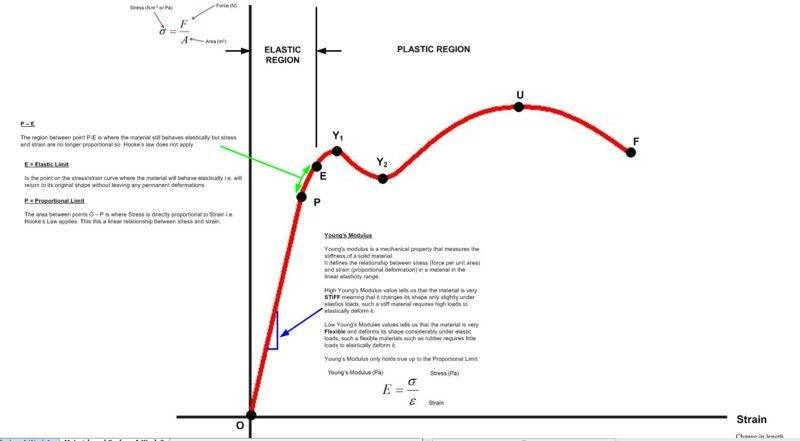# Difference between Elastic Limit & Yield Point

Hi All

I am trying to understand a stress / strain curve for a ductile material.

But I am struggling with understanding the difference between the Elastic Limit and the Yield Point. I define these terms as:-

Elastic Limit - Is the point on the stress/strain curve where the material will behave elastically i.e. will return to its original shape without leaving any permanent deformations.

Yield Point - The yield point is the point on a stress–strain curve that indicates the limit of elastic behaviour and the beginning of plastic behaviour.

Assuming that you agree with these definitions then the Elastic limit and Yield Point should be the same point on the stress strain curve - But they are NOT???

To help me better understand I created a stress strain curve for a ductile material and labelled them.I understand everything up to point E which is the elastic limit.

I have been told that Y1 and Y2 are yield points but why are there two of them and why is E and Y1 on different points on the curve?

What is happening between E and Y1?

Can anyone help explain?

Thank you.

## Answers and Replies

CWatters
Science Advisor
Homework Helper
Gold Member
I might be wrong but I thought the yield point was the point at which strain started to increase without much or any extra stress being added. So ductile materials have wider region between the two points than brittle materials.

jrmichler
Mentor
Some materials do not have a well defined yield point, so the yield point is defined as 0.2% plastic strain. That could be point E on your curve. Points Y1 and Y2 apply to some (not all) ductile materials, where the stress decreases immediately after yielding. They are the upper and lower yield points. So "the" yield point could be point E, point Y1, or somewhere between those two points.

Wikipedia has a good article: https://en.wikipedia.org/wiki/Yield_(engineering)